Square Roots 9
Set 9 (10 Questions)
This set has higher values to simplify than Set 8.
Students use exponents, powers, and roots, and use exponents in working with fractions.
Use square root and cube root symbols to represent solutions to equations of the form x-squared=p and x-cubed=p, where p is a positive rational number. Evaluate square roots of small perfect squares and cube roots of small perfect cubes. Know that the square root of 2 is irrational.

From Mr. Anker Tests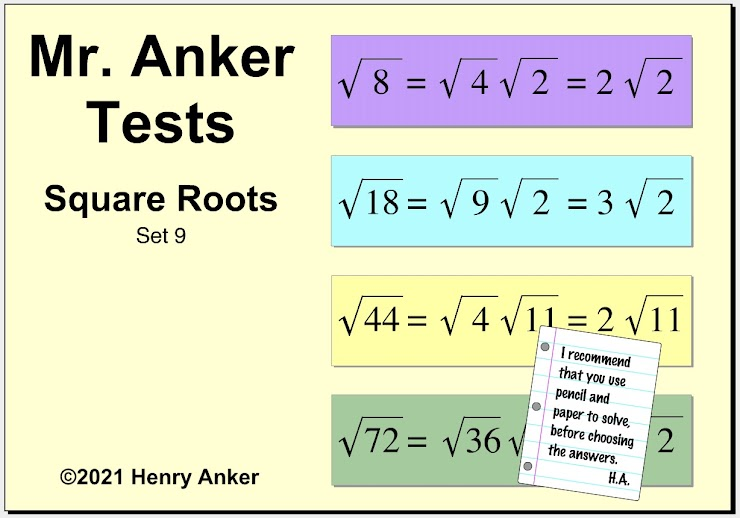Square Roots Help Slide 1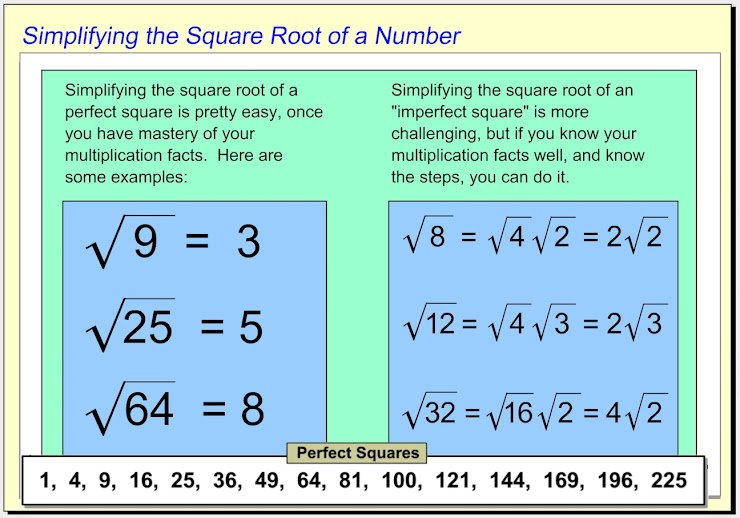Square Roots Help Slide 2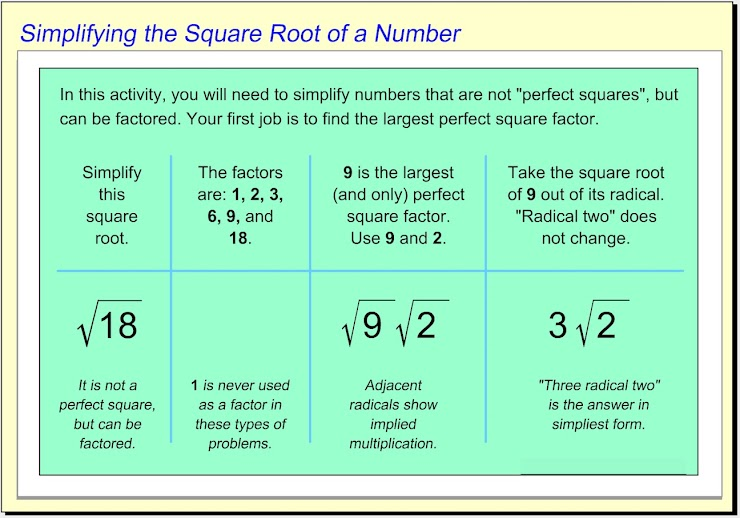Square Roots Help Slide 3Square Roots Help Slide 4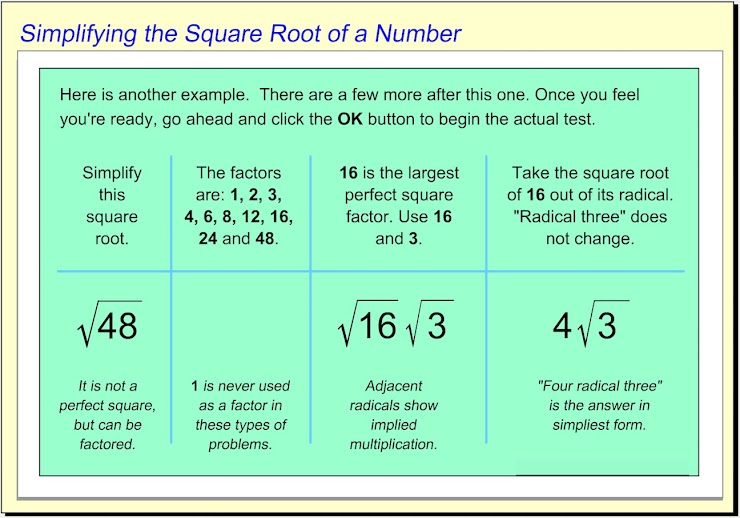Square Roots Help Slide 5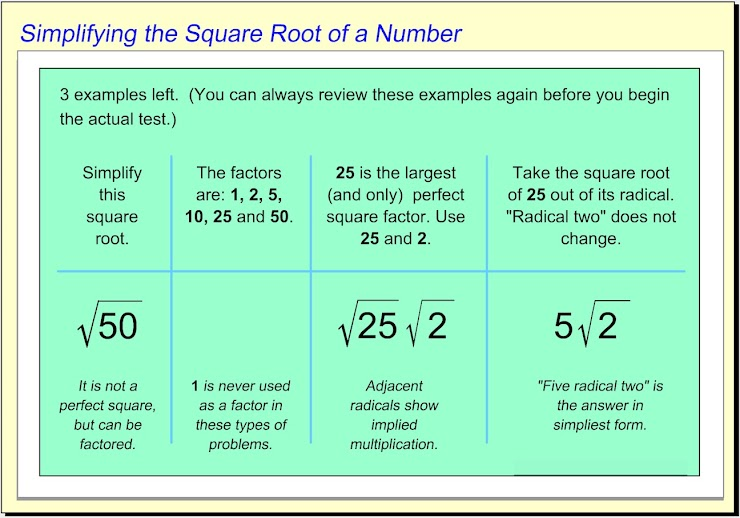Square Roots Help Slide 6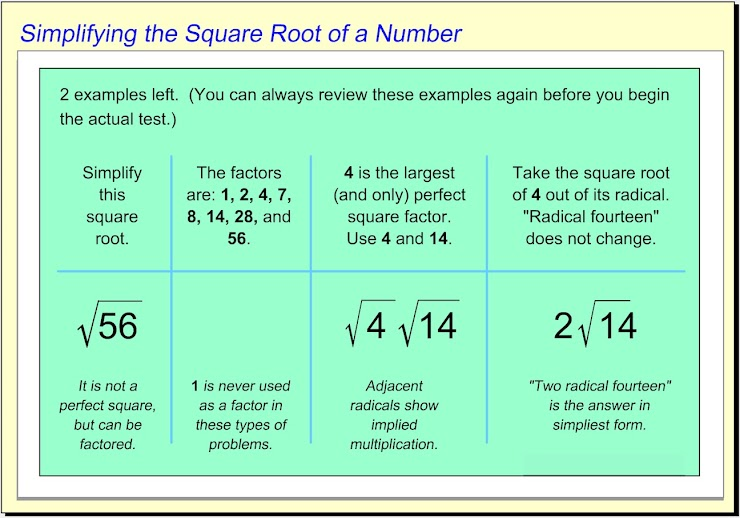Square Roots Help Slide 7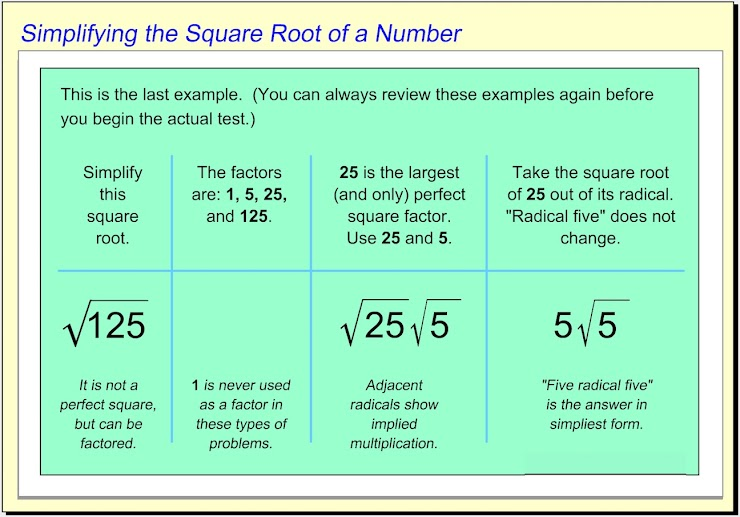1.  Which choice shows the simplification of the square root displayed? *
1 point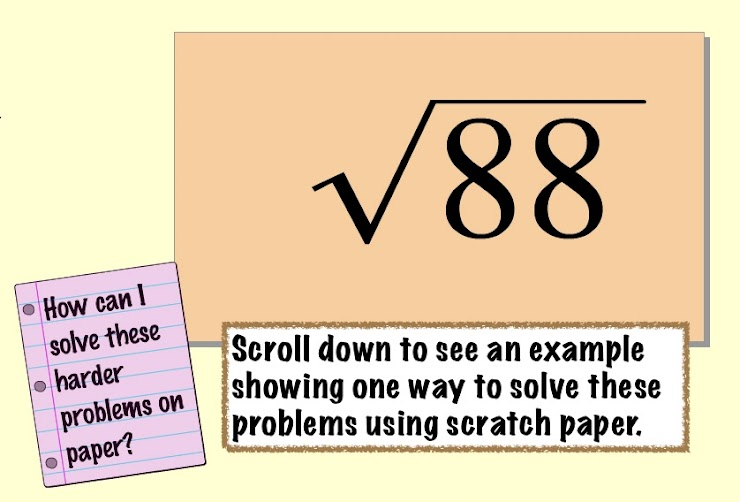Sample method for simplifying square roots...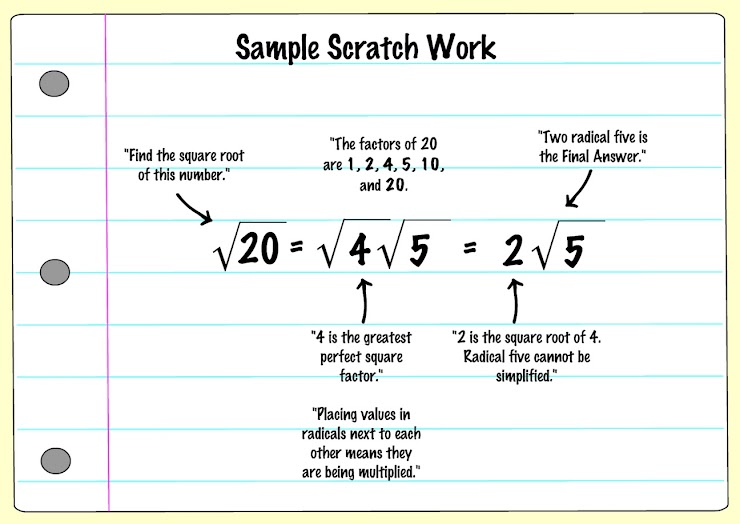2.  Which choice shows the simplification of the square root displayed? *
1 point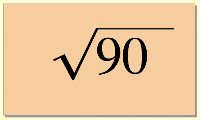3. Which choice shows the simplification of the square root displayed? *
1 point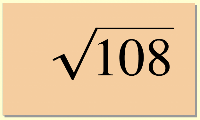4. Which choice shows the simplification of the square root displayed? *
1 point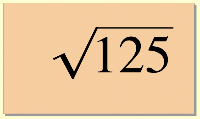5.  Which choice shows the simplification of the square root displayed? *
1 point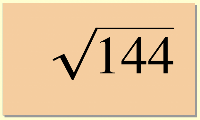6.  Which choice shows the simplification of the square root displayed? *
1 point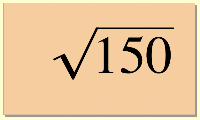7.  Which choice shows the simplification of the square root displayed? *
1 point8.  Which choice shows the simplification of the square root displayed? *
1 point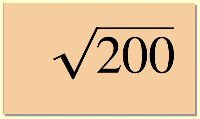9.  Which choice shows the simplification of the square root displayed? *
1 point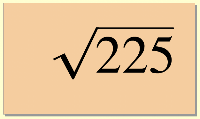10.  Which choice shows the simplification of the square root displayed? *
1 point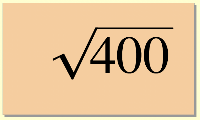Submit
Clear form